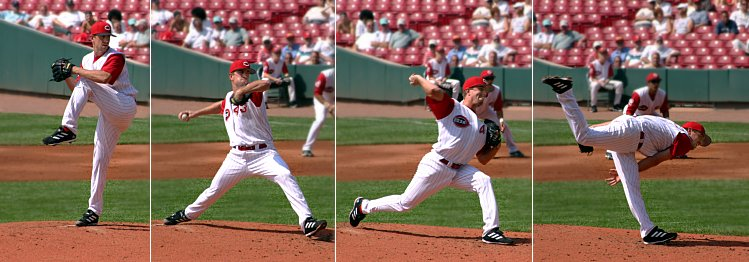# Momentum

Momentum is a property of an object's motion. When a push or a pull (a force) acts on an object and changes its motion, the quantity that gets changed is momentum. Energy is required to change the magnitude (size) of momentum, but not its direction. Specifically, momentum (written as ) is the mass of the object times its velocity (or speed with direction):

A force exerted for an amount of time gives a change in momentum (a force exerted over a distance is work instead of a change in momentum):

Massive objects have more momentum for a given speed, while lighter objects have less momentum. This is why it takes more effort (force) to stop a fully loaded truck than an empty one. Likewise, faster moving objects have more momentum than slower moving objects.

One way to express kinetic energy is in terms of momentum ():Figure 1. A pitcher exerts a force on a baseball in order to increase its momentum. His arm goes back as far as possible and then as forward as possible in order to maximize the time the force was applied.